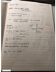# MATA32H3 Lecture Notes - Net Present Value, Cash Flow, Compound Interest

46 views7 pages
School
Department
Coursemehergurbani03 and 39451 others unlocked53
Verified Note
53 documents

## Document Summary

Main formula for compound interest is on p. 209. S=compound interest r=periodic interest rate (amount of interest paid per compounded period) n=number of compounding periods (number of time the interest is paid) If interest is paid every month it would be 12. We"re often given an apr (annual % rate of interest) and a frequency (number of times interest is paid per year, number of compoundings per year). k= frequency a= apr. R= when t is # of years, we have that n=kt. We have this expanded compound and formula. Ex1) you invest k @ 3. 05% apr compounding monthly for 5 years: find the compound amount, s. We solve for t in the following equation: ln key (log key) So, we need 13 years and 4 months. (p>0), (a>0, a decimal), k in. Ex2) [example on doubling time , p. 210 ex. 2&6] we invest at an apr compounding k times a year.